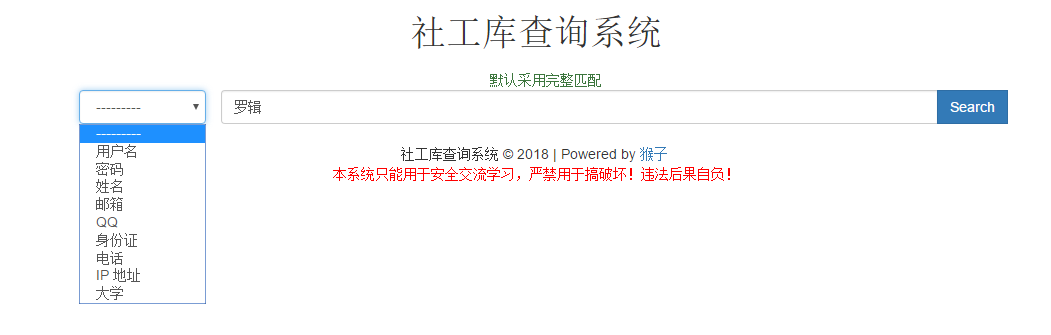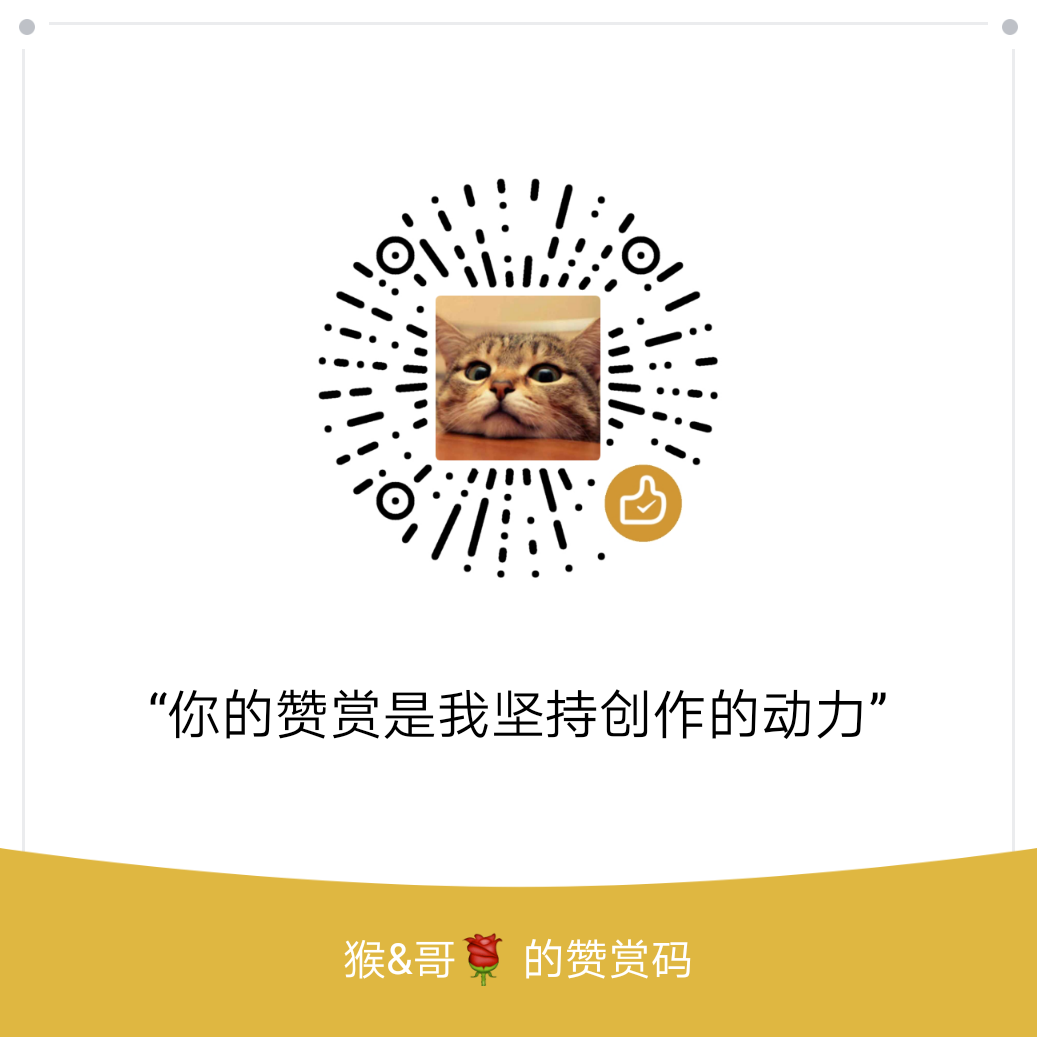• 欢迎访问极客猴，分享 Python 入门，网络爬虫，数据分析，赚钱思维相关的技术与思维`QQ群`
• 本站点文章首发于微信公众号【极客猴】，欢迎关注，第一时间接受文章推送~
• 如果您觉得本站非常有看点，那么赶紧使用 Ctrl+D 收藏极客猴吧

# Django 实战1：搭建属于自己社工查询系(上)

3年前 (2018-04-07) 19次浏览

## 2.数据库表

``````Create table if not exists `SocialUsers` (
`id` INTEGER PRIMARY KEY,
`chineseName` varchar(64) ,
`email` varchar(64) ,
`QQ` varchar(15) ,
`weibo` varchar(300),
`identity_number` varchar(25),
`cell_phone` varchar(20),
`college` varchar(60),
`living_place` varchar(200),
`source` varchar(50),
`remarks` varchar(400)
);``````## 3.模型建立

``````class Socialusers(models.Model):
id = models.IntegerField(blank=True, primary_key=True)
chinesename = models.CharField(db_column='chineseName', max_length=64, blank=True, null=True)
email = models.CharField(max_length=64, blank=True, null=True)
qq = models.CharField(db_column='QQ', max_length=15, blank=True, null=True)
weibo = models.CharField(max_length=300, blank=True, null=True)
identity_number = models.CharField(max_length=25, blank=True, null=True)
cell_phone = models.CharField(max_length=20, blank=True, null=True)
college = models.CharField(max_length=60, blank=True, null=True)
living_place = models.CharField(max_length=200, blank=True, null=True)
source = models.CharField(max_length=50, blank=True, null=True)
remarks = models.CharField(max_length=400, blank=True, null=True)

class Meta:
managed = True
db_table = 'SocialUsers'``````

## 4.表单实现

### 4-1.根据 model 生成 Form

``````# forms.py
CONDITION_CHOICES = (
('chineseName', '姓名'),
('email', '邮箱'),
('QQ', 'QQ'),
('identity_number', '身份证'),
('cell_phone', '电话'),
('college', '大学'),
('source', '来源'),
)

class QueryUserForm(forms.Form):
condition = forms.CharField(  # 也可以定义为 ChoiceField
max_length=20,
widget=forms.Select(choices=CONDITION_CHOICES,
attrs={'class':"form-control",
'title':"query condition",
'name':'condition',
}),
)

queryContent = forms.CharField(
max_length=100,
widget=forms.TextInput(attrs={'class': 'form-control is-invalid',
'name': 'queryContent',
'placeholder': '请输入需要要查询的内容...'
}),
error_messages={'required': '查询内容不能为空 !'}
)``````

### 4-2.自定义 Form

``````# models.py
# 用于表单查询的 model
CONDITION_CHOICES = (
('chineseName', '姓名'),
('email', '邮箱'),
('QQ', 'QQ'),
('identity_number', '身份证'),
('cell_phone', '电话'),
('college', '大学'),
('source', '来源'),
)

class QueryUser(models.Model):
condition = models.CharField(max_length=20, choices=CONDITION_CHOICES)
queryContent = models.CharField(max_length=100)

def __str__(self):    # __unicode__ on Python 2
return self.condition``````

Form 的实现代码如下：

``````# forms.py
class QueryUserForm(ModelForm):
class Meta:
model = QueryUser
fields = ['condition', 'queryContent', ]
# 指定呈现样式字段、指定 CSS 样式
widgets = {
'condition': Select(attrs={'class':"form-control",
'title':"query condition",
'name':'condition',
}),
'queryContent':TextInput(attrs={'class': 'form-control is-invalid',
'name': 'queryContent',
'placeholder': '请输入需要要查询的内容...'
})
}

localized = {
'queryContent':123
}

# 自定义错误信息
error_messages = {
'queryContent':{
'required': '查询内容不能为空 !',
}
}``````

## 5.模板创建

``````{% load staticfiles %}
<!DOCTYPE html>
<html>
<body>
<div class="container" id="container">
<h1 class="text-center"> 社工库查询系统 </h1>
</div>

<div class="row">
<form action="" method="GET" class="form-horizontal" role="form">
<div id="checkbox" class="text-center">
<label class="checkbox-inline text-success">默认采用完整匹配</label>
</div>

<div class="col-md-10 col-md-offset-1">
<div class="col-md-2 col-md-offset-0">
{{ form.condition }}
{% comment %}
{{ form.condition }} 在 html 中将被渲染成以下代码
<select name='condition' title="query condition" class="form-control">
<option >用户名</option>
<option>密码</option>
<option>姓名</option>
<option>邮箱</option>
<option>QQ</option>
<option>身份证</option>
<option>电话</option>
<option>大学</option>
<option>来源</option>
</select>
{% endcomment %}
</div>

<div class="input-group col-md-10 col-md-offset-1">
{{ form.queryContent.field.errors }}
{{ form.queryContent }}

{% comment %}
{{ form.queryContent }} 在 html 中将被渲染成以下代码
<input type="text" class="form-control is-invalid" name="q" placeholder="请输入内容..." value="">
{% endcomment %}

<div class="input-group-btn">
<button type="submit" class="btn btn-primary" required >Search</button>
<div class="invalid-feedback">
</div>
</div>
</div>
</div>
</form>
</div>
<br>
</div>
</body>
</html>``````

## 6.视图

``````# views.py
def index(request):
templateView = 'index.html'

if request.method == 'GET':
form = QueryUserForm(request.GET)
# 验证表单
if form.is_valid():
# 过滤需要的数据, 获取查询条件和查询内容
condition = form.cleaned_data['condition']
keywords = form.cleaned_data['queryContent']
print('condition == ' + condition)
print('keywords == ' + keywords)

# 查询数据...

return render(request, templateView, {'form': form})
# 直接访问主页, 显示的内容
else:
return render(request, templateView, {'form': form})``````

## 7.源码[赞赏码]